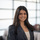# [Solution] Divide by Zero

## Click here to view the question

Solution:

```select
platform
, case
when total_users = 0
then null
else active_users * 1.0 / total_users
end as percent_active_users
from
platform_summary
```

Concepts Covered: SQL Division, Case When Statements

Explanation:

We write a case when statement to check if the denominator is 0. If it is, we return a null result, else we perform the division as normal. Note that we multiply the numerator by 1.0 as we are dividing 2 integers and looking for a float output.